# Selina solutions for Concise Physics Class 7 ICSE chapter 4 - Light Energy [Latest edition]

#### Chapters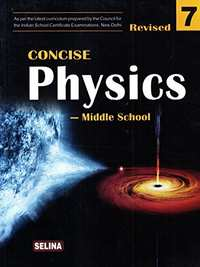## Chapter 4: Light Energy

Objective QuestionsShort/Long Answer Questions
Objective Questions

### Selina solutions for Concise Physics Class 7 ICSE Chapter 4 Light Energy Objective Questions

Objective Questions | Q 1.01

Write true or false of the following statement.

The image formed by a plane mirror is real

• True

• False

Objective Questions | Q 1.02

Write true or false of the following statement.

When a light ray is reflected from a wall, the angle of incidence is not equal to the angle of reflection.

• True

• False

Objective Questions | Q 1.03

Write true or false of the following statement.

The image of the right hand in a plane mirror looks like that of a left hand.

• True

• False

Objective Questions | Q 1.04

Write true or false of the following statement.

The image formed by a plane mirror is upright.

• True

• False

Objective Questions | Q 1.05

Write true or false of the following statement.

The image formed by a plane mirror can be obtained on a screen.

• True

• False

Objective Questions | Q 1.06

Write true or false of the following statement.

The objects are seen around us due to irregular reflection of light.

• True

• False

Objective Questions | Q 1.07

Write true or false of the following statement.

The speed of light in vacuum is 3 × 108 ms-1.

• True

• False

Objective Questions | Q 1.08

Write true or false of the following statement.

A rose appears red in light of all the colours.

• True

• False

Objective Questions | Q 1.09

Write true or false of the following statement.

A black paper absorbs light of all the colours and reflects none.

• True

• False

Objective Questions | Q 1.1

Write true or false of the following statement.

The primary colours are red, blue and green.

• True

• False

Objective Questions | Q 2.01

Fill in the blank

Angle of incidence = ___________

Objective Questions | Q 2.02

Fill in the blank

The incident ray, the reflected ray and the normal lie in one _______

Objective Questions | Q 2.03

Fill in the blank

The image formed by a plane mirror is at a distance behind the mirror as ________

Objective Questions | Q 2.04

Fill in the blank

The image formed by a plane mirror is erect and _____

Objective Questions | Q 2.05

Fill in the blank

We are able to see the objects around us due to _________, reflection.

Objective Questions | Q 2.06

Fill in the blank

A ________ image cannot be obtained on a screen.

Objective Questions | Q 2.07

Fill in the blank

A plane mirror _________ reflects 100 percent light falling on it.

Objective Questions | Q 2.08

Fill in the blank

The colour of an opaque object is the colour of light which it _______

Objective Questions | Q 2.09

Fill in the blank

Magenta, cyan and yellow are the ________ colours.

Objective Questions | Q 3

Match the following

 (a). A light ray passes             from air to glass (1) . speeds up (b). A light ray passes             from glass to water (2). reflects red light (c). Virtual image (3). primary colours (d). Red rose (4). plane mirror (e). Red, green, and               blue (5). slows down
Objective Questions | Q 4.01

Select the correct alternative

A man standing in front of a plane mirror finds his image to be at a distance of 6 meter from himself. The distance of man from the mirror is

• 6 m

• 3 m

• 2 m

• 12 m

Objective Questions | Q 4.02

Select the correct alternative

The angle between the incident ray and the ray reflected from the plane mirror is 70°. The angle of incidence will be :

• 70°

• 30°

• 35°

• 90°

Objective Questions | Q 4.03

Select the correct alternative

The image formed by a plane mirror is

• virtual and inverted

• virtual and of same size

• real and inverted

• real and of same size

Objective Questions | Q 4.04

Select the correct alternative

The angle of incidence on a plane mirror is 30°.The angle of reflection will be:

• 30°

• 60°

• 15°

• 0°

Objective Questions | Q 4.05

Select the correct alternative

The angle of incidence on a plane mirror is 30°. The angle between the incident ray and the reflected ray is

• 30°

• 15°

• 60°

• 90°

Objective Questions | Q 4.06

Select the correct alternative

The property due to which a light ray striking a surface is returned back into the same medium is called

• refraction

• reflex action

• reflection

• regression

Objective Questions | Q 4.07

Select the correct alternative

The speed of light is maximum in

• glass

• water

• air

• wood

Objective Questions | Q 4.08

Select the correct alternative

A red rose is seen in green light. It will appear.

• red

• blue

• yellow

• black

Objective Questions | Q 4.09

Select the correct alternative

The primary colours are

• Red, Blue and Yellow

• Magenta, Yellow and Cyan

• Red, Blue and Cyan

• Blue, Green and Red

### Selina solutions for Concise Physics Class 7 ICSE Chapter 4 Light Energy Short/Long Answer Questions

Short/Long Answer Questions | Q 1

What do you mean by the term reflection of light ?

Short/Long Answer Questions | Q 2

How is a plane mirror made ?

Short/Long Answer Questions | Q 3

Explain the following terms:

Incident ray, Reflected ray, Angle of incidence, Angle of reflection, Normal.

Short/Long Answer Questions | Q 4

Draw a diagram showing the reflection of a light ray from a plane mirror. Label on it the incident ray, the reflected ray, the normal, the angle of incidence i and the angle of reflection r.

Short/Long Answer Questions | Q 5

State the two laws of reflection of light.

Short/Long Answer Questions | Q 6

Describe an experiment to verify the laws of reflection of light. Ans. Laws of reflection.

Short/Long Answer Questions | Q 7

A ray of light falls normally on a plane mirror. What is the angle of incidence ?

Short/Long Answer Questions | Q 8

Draw a diagram to show the reflection of a light ray incident normally on a plane mirror.

Short/Long Answer Questions | Q 9

The diagram in Fig. shows an incident ray AO and the reflected ray OB from a plane mirror. The angle AOB is 30°. Draw normal on the plane mirror at the point O and find :

Short/Long Answer Questions | Q 10.1

In the following diagrams, measure and write the angle of incidence and draw the reflected ray in given case.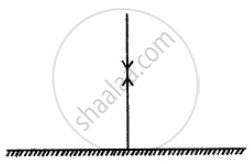Short/Long Answer Questions | Q 10.2

In the following diagrams, measure and write the angle of incidence and draw the reflected ray in each case.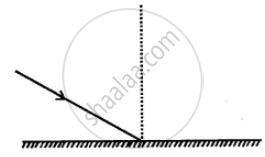Short/Long Answer Questions | Q 10.3

In the following diagrams, measure and write the angle of incidence and draw the reflected ray in each case.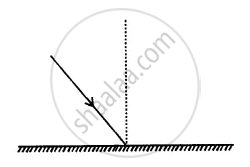Short/Long Answer Questions | Q 11

The diagram in fig. shows an incident ray AO and the normal ON on a plane mirror. Draw the reflected ray. State the law you use to draw the direction of the reflected ray.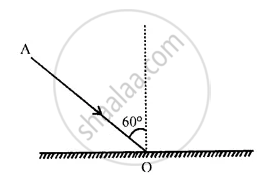Short/Long Answer Questions | Q 12

The following diagram shows an incident ray AO and the normal ON on a plane mirror. Find the angle of incidence and angle of reflection.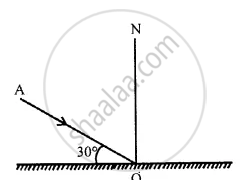Short/Long Answer Questions | Q 13

State in words, how do you find the location of image of an object formed by a plane mirror.

Short/Long Answer Questions | Q 14

Draw a ray diagram showing the formation of image of a point object by a plane mirror.

Short/Long Answer Questions | Q 15

The following diagram shows a point object O placed in front of a plane mirror. Take two rays from the point O and show how the image of O is formed and seen by the eye.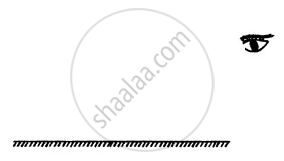Short/Long Answer Questions | Q 16

State four characteristics of the image formed by a plane mirror.

Short/Long Answer Questions | Q 17

How is the position of image formed by a plane mirror related to the position of the object ?

Short/Long Answer Questions | Q 18.1

You are standing at a distance of 2 meters from a plane mirror.

What is the distance of your image from the mirror?

Short/Long Answer Questions | Q 18.2

You are standing at a distance of 2 meters from a plane mirror.

What is the distance between you and your image?

Short/Long Answer Questions | Q 19

What is meant by lateral inversion of an image in a plane mirror ? Explain it with the help of a diagram.

Short/Long Answer Questions | Q 20

Wirte down the letter C and I as seen in a plane mirror.

Short/Long Answer Questions | Q 21

What is irregular reflection ? Give an example.

Short/Long Answer Questions | Q 22

How do we see objects around us ?

Short/Long Answer Questions | Q 23

State two uses of a plane mirror.

Short/Long Answer Questions | Q 24

Can light travel in vacuum ?

Short/Long Answer Questions | Q 25

State the speed of light in (a) air, (b) glass.

Short/Long Answer Questions | Q 26.1

State whether light slows down or speeds up in the following cases :

Light going from air to glass.

Short/Long Answer Questions | Q 26.2

State whether light slows down or speeds up in the following cases :

Light going from glass to water.

Short/Long Answer Questions | Q 26.3

State whether light slows down or speeds up in the following cases :

Light going from water to air.

Short/Long Answer Questions | Q 27

What are the primary colours ? Name the three primary colours.

Short/Long Answer Questions | Q 28

What are the secondary colours ? Name the three secondary colours.

Short/Long Answer Questions | Q 29.1

Fill in the blank with the appropriate colour

Blue + _______ = Cyan

Short/Long Answer Questions | Q 29.2

Fill in the blank with the appropriate color

Red + Blue + _______ = White

Short/Long Answer Questions | Q 29.3

Fill in the blank with the appropriate colour

Red + Blue = _______

Short/Long Answer Questions | Q 29.4

Fill in the blank with the appropriate colour

Green + Red = ______

Short/Long Answer Questions | Q 30

The leaves appear green when seen in white light. Give a reason.

Short/Long Answer Questions | Q 31.1

A rose appears red in white light. How will it appear in
green light? Give a reason for your answer.

Short/Long Answer Questions | Q 31.2

A rose appears red in white light. How will it appear in red light?

Short/Long Answer Questions | Q 32

Why does a piece of paper appear white in sunlight ? How would you expect it to appear when viewed in red light?

Short/Long Answer Questions | Q 33

A piece of paper appears black in sunlight. What will be it£ colour when seen in red light ?

## Chapter 4: Light Energy

Objective QuestionsShort/Long Answer Questions## Selina solutions for Concise Physics Class 7 ICSE chapter 4 - Light Energy

Selina solutions for Concise Physics Class 7 ICSE chapter 4 (Light Energy) include all questions with solution and detail explanation. This will clear students doubts about any question and improve application skills while preparing for board exams. The detailed, step-by-step solutions will help you understand the concepts better and clear your confusions, if any. Shaalaa.com has the CISCE Concise Physics Class 7 ICSE solutions in a manner that help students grasp basic concepts better and faster.

Further, we at Shaalaa.com provide such solutions so that students can prepare for written exams. Selina textbook solutions can be a core help for self-study and acts as a perfect self-help guidance for students.

Concepts covered in Concise Physics Class 7 ICSE chapter 4 Light Energy are Reflection of Light, Terms Used in Reflection of Light, Law of Reflection of Light, Images Formed by a Plane Mirrors, Speed of Light, Colour, Light, Plane Mirror and Reflection, Verification of the Law of Reflection of Light, Reflection of a Ray of Light Normally Incident on a Plane Mirror, Formation of Image by Reflection: Real and Virtual Image, Lateral Inversion, Types of Reflection.

Using Selina Class 7 solutions Light Energy exercise by students are an easy way to prepare for the exams, as they involve solutions arranged chapter-wise also page wise. The questions involved in Selina Solutions are important questions that can be asked in the final exam. Maximum students of CISCE Class 7 prefer Selina Textbook Solutions to score more in exam.

Get the free view of chapter 4 Light Energy Class 7 extra questions for Concise Physics Class 7 ICSE and can use Shaalaa.com to keep it handy for your exam preparation